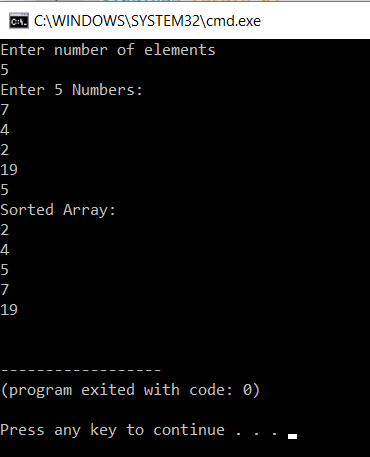# How To Implement Bubble Sort In C?

Last updated on Aug 08,2019 2.2K ViewsHow To Implement Bubble Sort In C?### myMock Interview Service for Real Tech Jobs### myMock Interview Service for Real Tech Jobs

• Mock interview in latest tech domains i.e JAVA, AI, DEVOPS,etc
• Get interviewed by leading tech experts
• Real time assessment report and video recording

Sorting data can be of utmost of importance. Especially in situations where you need data arranged in a specific order. In this article ‘Bubble Sorting In C‘ we would understand one of the popular sorting techniques in the market.

So let us get started then,

## Bubble Sort in C

Bubble sort is one of the easiest sorting techniques in programming and it is very simple to implement. It just simply compares the current element with the next element and swaps it, if it is greater or less, depending on the condition. It gives quite accurate results. Each time an element is compared with all other elements till it’s final place is found is called a pass.

Bubble sort gets its name because it filters out the elements at the top of the array like bubbles on water.

It is the slowest algorithm and it runs with a time complexity of O(n^2). Bubble sort can be optimized by using a flag variable that exits the loop once swapping is done. The best complexity of a bubble sort can be O(n). O(n) is only possible if the array is sorted.

Here is an example:

(6 4 2 1 3)

After you implement bubble sort, above array will be turned to (1 2 3 4 6)

Here is the example program:

```int main()
{
int array, n, i, j, swap;
printf("Enter number of elementsn");
scanf("%d", &n);
printf("Enter %d Numbers:n", n);
for(i = 0; i < n; i++)
scanf("%d", &array[i]);
for(i = 0 ; i < n - 1; i++)
{
for(j = 0 ; j < n-i-1; j++)
{
if(array[j] > array[j+1])
{
swap=array[j];
array[j]=array[j+1];
array[j+1]=swap;
}
}
}
printf("Sorted Array:n");
for(i = 0; i < n; i++)
printf("%dn", array[i]);
return 0;
}
```

Output:

This program gives you a demonstration of bubble sort algorithm. In the first part of the code we accept the number of terms in the array and store it in n. In the next part, the user enters the elements of the array.Then there are two ‘for loops’. The first ‘for loop’ runs from I value equal to zero all the way till it is less than n-1.  The outer array takes care of the element of the value to be compared to all the other elements.

Inside the for loop, there is another for loop that starts from j=0 all the way to j<n-i-1. This loop takes care of all elements. Inside, there is an if statement that compares if a[j]>a[j+1]. If this condition is satisfied then swapping takes place. A variable called swap is used for this. First, a[j] is assigned to swap, followed by a[j+1] being assigned at a[j] and at last swap is assigned to a[j+1]. This continues till all the elements are sorted. After this, the sorted array is printed.

This is how the bubble sort is done.

## Optimized Implementation of Bubble Sort

This is done to improve the results of bubble sort. To get an optimized output we insert a flag variable in the for loop. This variable will hold one if there is swapping. If not then it will break out of the for loop and save time.

```
#include <stdio.h>
int main()
{
int array, n, i, j, swap,flag=0;
printf("Enter number of elementsn");
scanf("%d", &n);
printf("Enter %d Numbers:n", n);
for(i = 0; i < n; i++)
scanf("%d", &array[i]);
for(i = 0 ; i < n - 1; i++)
{
for(j = 0 ; j < n-i-1; j++)
{
if(array[j] > array[j+1])
{
swap = array[j];
array[j] = array[j+1];
array[j+1] = swap;
flag=1;
}
if(!flag)
{
break;
}
}
}
printf("Sorted Array:n");
for(i = 0; i < n; i++)
printf("%dn", array[i]);
return 0;
}

```

Example:

`The execution of this program is similar to that of the normal bubble sort but, the only change is flag variable. The flag variable is set to one if there is a swap. This means that the array still requires more checking. If the flag is not 1 then we exit from the loop thinking that the array is already sorted.`

The output is the same

This brings us to the final part of this Bubble Sort in C

## Time Complexity

The best complexity of this algorithm is O(n). This is when the array is sorted.

The worst complexity in this algorithm is O(n*n). Here the array is not sorted.

With this we come to the end of this blog on ‘Bubble Sort In C’. I hope you found this informative and helpful, stay tuned for more tutorials on similar topics.You may also checkout our training program to get in-depth knowledge on jQuery along with its various applications, you can enroll here for live online training with 24/7 support and lifetime access.

Got a question for us? Mention them in the comments section of  this blog and we will get back to you.REGISTER FOR FREE WEBINARThank you for registering Join Edureka Meetup community for 100+ Free Webinars each month